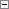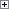C/AL contains several functions that can be used for different purposes, such as string handling, text formatting, database handling, and so on. Some of these functions can use different parameters.

Parameters

In a function call, the parameters are separated by commas, and the optional parameters may be omitted starting from the right. For example, this means that if a function has three optional parameters, then you cannot omit the second parameter without omitting the third parameter.

You can specify that a parameter is passed to a function by value or by reference.

• If a parameter is passed by value, then a copy of the variable is passed to the function. Any changes that the function makes to the value of the variable are local changes that affect only the copy, not the variable itself.
• If a parameter is passed by reference, then a reference to the variable is passed to the function. The function can change the value of the variable itself.

For more information about how to specify that a parameter is passed by value or by reference, see How to: Add a Function to a Codeunit.

Example 1

The following shows the syntax for a function.Copy Code
FUNCTION([Optional1] [, Optional2] [, Optional3])

The function that uses the syntax above can be called by using the following code.Copy Code
FUNCTION(Optional1, Optional2)

This function cannot be called by using the following code.Copy Code
FUNCTION(, Optional2, Optional3)

Example 2

ABS is an example of a C/AL function that has a fixed number of parameters (1).Copy Code
Value := -1033; //A negative integer value
PositiveValue := ABS(Value); //Calculate the positive value 1033

Example 3

The function DMY2DATE is an example of a function that can be called by using a variable number of parameters.Copy Code
NewDate := DMY2DATE(5, 11, 1992); //Returns the date November 5, 1992

Depending on the use of the DMY2DATE function, 1, 2, or 3 parameters can be passed to the function because the second and third parameters are optional. When the second and third parameters are not used, values from the system date are used as default.

Return Values

A function can return a value. For more information about how to specify that a function has a return value, see How to: Add a Function to a Codeunit.

Example 1

You can assign the return value of a function to a variable.Copy Code
ReturnVal := MyFunction(Param1);

Example 2

In this example, MyFunction returns a Boolean value. You can use the return value in a conditional statement.Copy Code
IF (MyFunction(Param1)) THEN
<Statement1>
ELSE
<Statement2>